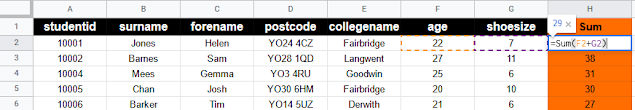## Tuesday 16 August 2022

The following Google Apps Script is designed to insert a formula into the first row of data in a Google Sheet, then use Autofill to add the formula to the subsequent rows below. In this example I am just using a simple Sum formula to add up age and shoesize for demo purposes. The need to do this came about when combining multiple Google Sheet files however, that needed calculations adding to the final composed version.Autofill Google Sheet Formula

### The Code

We start with the usual getting the current sheet, getting the last row of data (so we later know how far down to append to) and writing the formula we want to insert:

// get active sheet

// get last row of data for later pasting into
var lastRow = sheet.getLastRow();

// create Formula for first row
var formula = "=Sum(F2+G2)";

Next we get our source row (where the formula will first be inserted and then autofilled from). We get this as a separate variable because we will need to reference it more than once. We then apply our formula to this single row:

// get source range - where Formula will be inserted and autofilled from
var sourceRange = sheet.getRange(2, 8);

// paste Formula in to first row (ignoring Header row) aka source range
sourceRange.setFormula(formula);

Now that we have the formula in the Google Sheet we can make use of the autofill feature to apply it to the rest of our range:

// get the rest of the range to fill with the Formula (includes source range)
var destinationRange = sheet.getRange(2, 8, lastRow - 1, 1);

// insert Formula, continuing the pattern